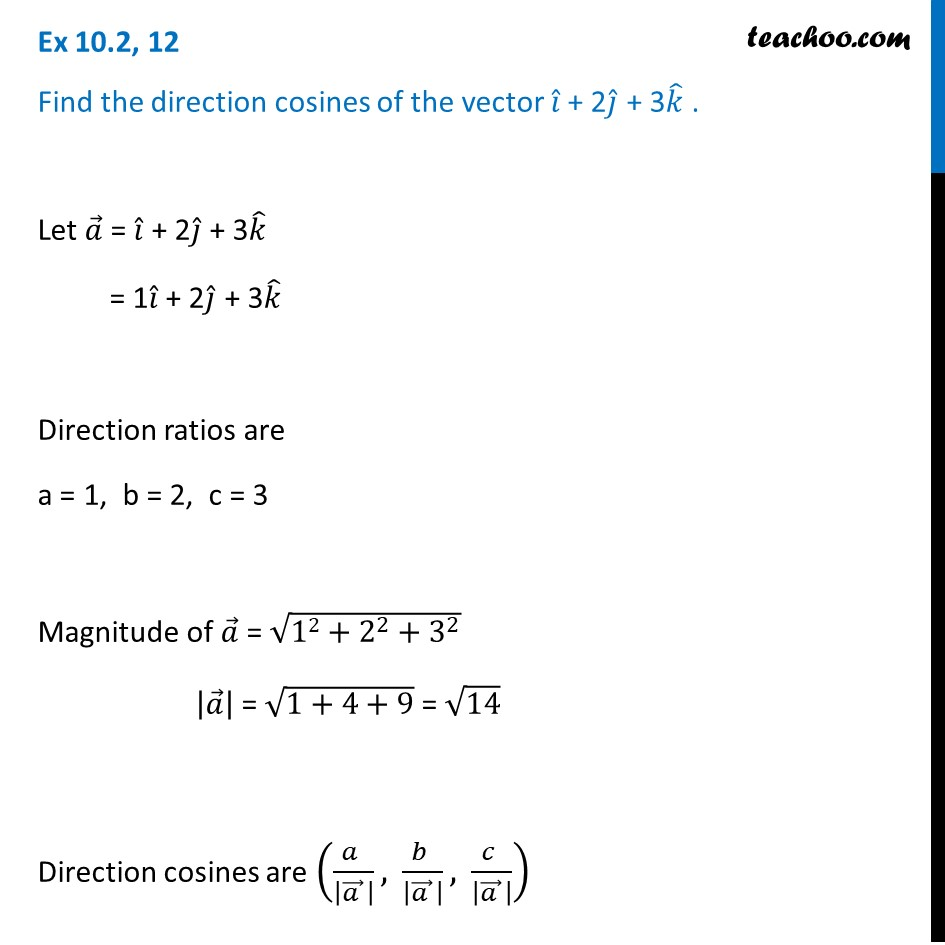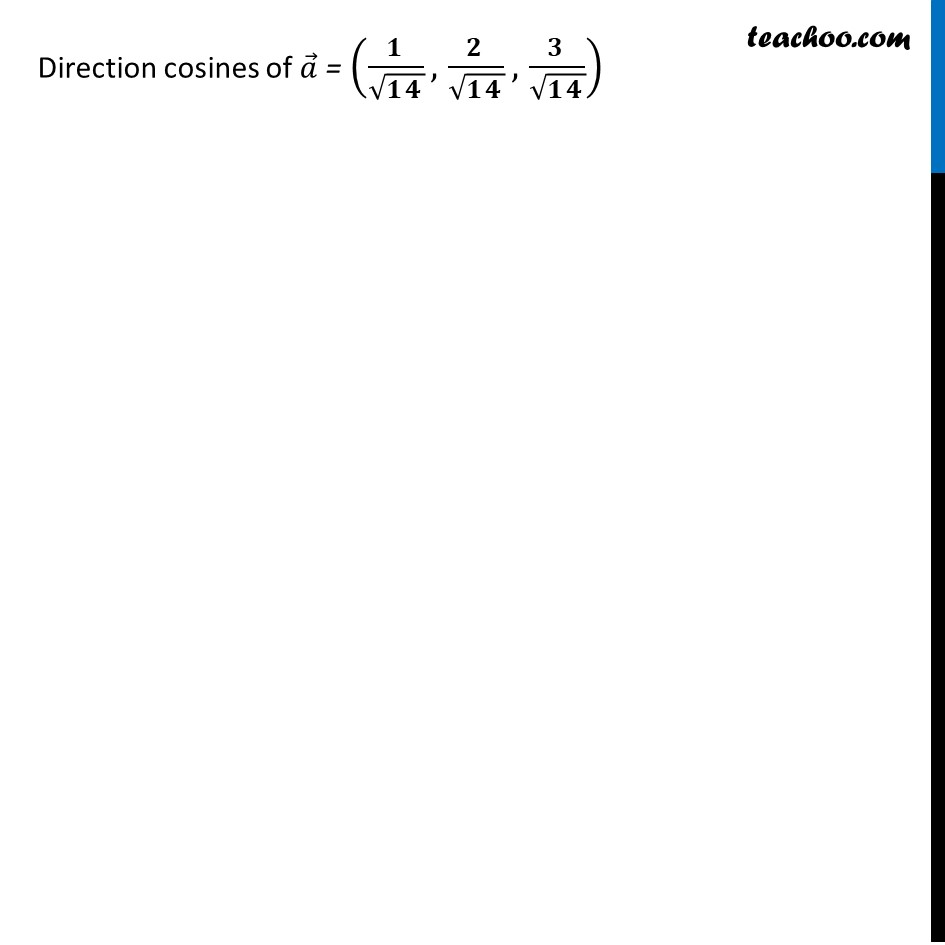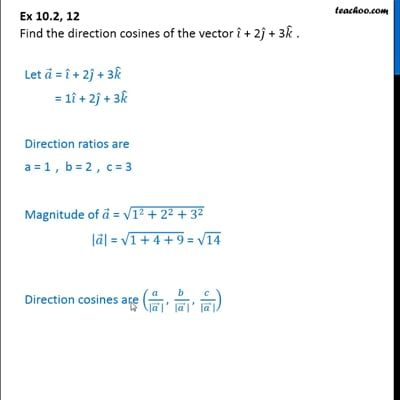Ex 10.2

Chapter 10 Class 12 Vector Algebra
Serial order wiseThis video is only available for Teachoo black users

Solve all your doubts with Teachoo Black (new monthly pack available now!)

### Transcript

Ex 10.2, 12 Find the direction cosines of the vector 𝑖 ̂ + 2𝑗 ̂ + 3𝑘 ̂ . Let 𝑎 ⃗ = 𝑖 ̂ + 2𝑗 ̂ + 3𝑘 ̂ = 1𝑖 ̂ + 2𝑗 ̂ + 3𝑘 ̂ Direction ratios are a = 1, b = 2, c = 3 Magnitude of 𝑎 ⃗ = √(12+2^2+3^2 ) |𝑎 ⃗ | = √(1+4+9) = √14 Direction cosines are (𝑎/|𝑎 ⃗ | ,𝑏/|𝑎 ⃗ | ,𝑐/|𝑎 ⃗ | ) Direction cosines of 𝑎 ⃗ = (𝟏/√𝟏𝟒,𝟐/√𝟏𝟒,𝟑/√𝟏𝟒)